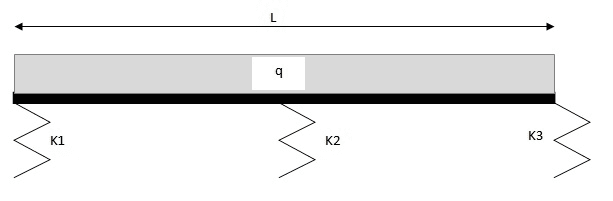# Undetermined beam problem supported by three different springs

• Eran
In summary,The beam in the OP rests on several discrete springs. There are other types of elastic support, like the one referenced in the article above, where a beam rests on a continuous elastic foundation of indeterminate length. I would think that analyzing the latter would be somewhat different than analyzing the former.Depending on the stiffness of the springs, have you considered analyzing the problem as if the beam were rigid and only the springs deflected?

#### Eran

Hi,

I thought it would be an easy one but it isn't...

I am trying to solve the problem of a beam supported by three different springs with a uniform distributed load:Eran said:
Hi,

I thought it would be an easy one but it isn't...

I am trying to solve the problem of a beam supported by three different springs with a uniform distributed load:

View attachment 99729
It doesn't matter if the supports are rigid or if the supports are springs: you have a beam which is statically indeterminate. You'll need to use more than the two equations of static equilibrium to obtain the reactions in each spring.

Thanks. But if I ignore the springs elasticity and just calculate the deflection between the supports I now need to add the deflection in each of the supporting points (because they are elastic). I can't just calculate the deflection of each point due to the calculated reaction because the reaction itself depends on the spring coefficient, doesn't it?

Eran said:
Thanks. But if I ignore the springs elasticity and just calculate the deflection between the supports I now need to add the deflection in each of the supporting points (because they are elastic). I can't just calculate the deflection of each point due to the calculated reaction because the reaction itself depends on the spring coefficient, doesn't it?
Correct.

There are methods for analyzing beams on elastic supports, just like there are methods for analyzing beams on fixed supports.

The following article includes (p. 5) the case of a cantilever beam propped on a spring at the free end:

Thanks. I know this article, but it isn't enough. Because when you have more than a single spring in the system there is also a rigid body movement of the beam involved and this somehow makes the solution much less intuitive and complex. There are articles on a non-uniform, flexible foundations (See http://boundaryvalueproblems.springeropen.com/articles/10.1186/1687-2770-2012-5) but those are way too complex for a simple problem like this one.

Eran said:
Thanks. I know this article, but it isn't enough. Because when you have more than a single spring in the system there is also a rigid body movement of the beam involved and this somehow makes the solution much less intuitive and complex. There are articles on a non-uniform, flexible foundations (See http://boundaryvalueproblems.springeropen.com/articles/10.1186/1687-2770-2012-5) but those are way too complex for a simple problem like this one.
The beam in the OP rests on several discrete springs. There are other types of elastic support, like the one referenced in the article above, where a beam rests on a continuous elastic foundation of indeterminate length. I would think that analyzing the latter would be somewhat different than analyzing the former.

Depending on the stiffness of the springs, have you considered analyzing the problem as if the beam were rigid and only the springs deflected?

Eran said:
Hi,

I thought it would be an easy one but it isn't...

I am trying to solve the problem of a beam supported by three different springs with a uniform distributed load:

View attachment 99729
You can try to solve this beam if you know the spring constants for the supports by using superposition.

This article has an example of a 2-span continuous beam where the reactions are calculated using superposition:

See pp. 8-9.

These problems are tough to solve in general. It's better if you have some specific springs in mind for the supports.

Mech_Engineer said:
Depending on the stiffness of the springs, have you considered analyzing the problem as if the beam were rigid and only the springs deflected?
Hi,

Yes. This is an easy task to do, but gets much more complicated when the beam is not considered as a rigid body

Are the springs the same order of magnitude in stiffness as the beam itself? It seems that if the beam is much stiffer than the springs (or if the springs are much stiffer than the beam) you should be able to solve via superposition...

Eran said:
Hi,

Yes. This is an easy task to do, but gets much more complicated when the beam is not considered as a rigid body
AFAIK, superposition does not assume the beam is a rigid body. In the example cited, the deflection of the beam due to the central support is calculated such that it exactly counteracts the central deflection of a simply supported beam with the distributed load shown.

Regarding superposition, I was referring to the possibility of solving the problems independently and then combining their results. This assumes the combined problems have minimal "interaction" as in the case the beam's stiffness is much different than the spring stiffness. It might be an approximation but could give a useful result.

Hi, I am currently working on a very similar problem and was curious if you ever solved it.